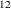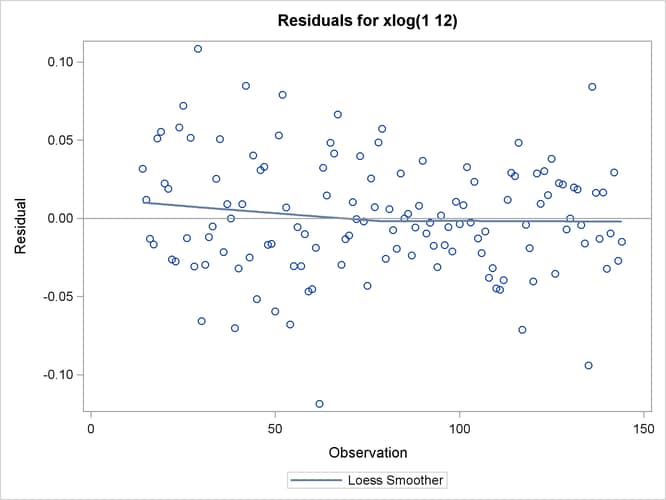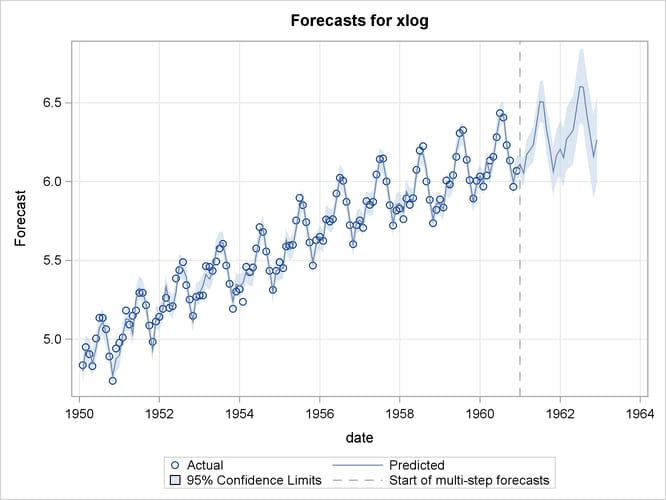# The ARIMA Procedure

### Statistical Graphics

Subsections:

Statistical procedures use ODS Graphics to create graphs as part of their output. ODS Graphics is described in detail in Chapter 21: Statistical Graphics Using ODS in SAS/STAT 13.2 User's Guide.

Before you create graphs, ODS Graphics must be enabled (for example, with the ODS GRAPHICS ON statement). For more information about enabling and disabling ODS Graphics, see the section "Enabling and Disabling ODS Graphics" in that chapter.

The overall appearance of graphs is controlled by ODS styles. Styles and other aspects of using ODS Graphics are discussed in the section "A Primer on ODS Statistical Graphics" in that chapter.

This section provides information about the graphics produced by the ARIMA procedure. (See Chapter 21: Statistical Graphics Using ODS in SAS/STAT 13.2 User's Guide, for more information about ODS statistical graphics.) The main types of plots available are as follows:

• plots useful in the trend and correlation analysis of the dependent and input series

• plots useful for the residual analysis of an estimated model

• forecast plots

You can obtain most plots relevant to the specified model by default. For finer control of the graphics, you can use the PLOTS= option in the PROC ARIMA statement. The following example is a simple illustration of how to use the PLOTS= option.

#### Airline Series: Illustration of ODS Graphics

The series in this example, the monthly airline passenger series, is also discussed later, in Example 7.2.

The following statements specify an ARIMA(0,1,1)(0,1,1)model without a mean term to the logarithms of the airline passengers series, xlog. Notice the use of the global plot option ONLY in the PLOTS= option of the PROC ARIMA statement. It suppresses the production of default graphics and produces only the plots specified by the subsequent RESIDUAL and FORECAST plot options. The RESIDUAL(SMOOTH) plot specification produces a time series plot of residuals that has an overlaid loess fit; see Figure 7.21. The FORECAST(FORECAST) option produces a plot that shows the one-step-ahead forecasts, as well as the multistep-ahead forecasts; see Figure 7.22.

proc arima data=seriesg
plots(only)=(residual(smooth) forecast(forecasts));
identify var=xlog(1,12);
estimate q=(1)(12) noint method=ml;
forecast id=date interval=month;
run;


Figure 7.21: Residual Plot of the Airline ModelFigure 7.22: Forecast Plot of the Airline Model#### ODS Graph Names

PROC ARIMA assigns a name to each graph it creates by using ODS. You can use these names to reference the graphs when you use ODS. The names are listed in Table 7.13.

Table 7.13: ODS Graphics Produced by PROC ARIMA

ODS Graph Name

Plot Description

Option

SeriesPlot

Time series plot of the dependent series

SeriesACFPlot

Autocorrelation plot of the dependent series

SeriesPACFPlot

Partial-autocorrelation plot of the dependent series

SeriesIACFPlot

Inverse-autocorrelation plot of the dependent series

SeriesCorrPanel

Series trend and correlation analysis panel

Default

CrossCorrPanel

Cross-correlation plots, either individual or paneled. They are numbered 1, 2, and so on as needed.

Default

ResidualACFPlot

Residual-autocorrelation plot

ResidualPACFPlot

Residual-partial-autocorrelation plot

ResidualIACFPlot

Residual-inverse-autocorrelation plot

ResidualWNPlot

Residual-white-noise-probability plot

ResidualHistogram

Residual histogram

ResidualQQPlot

Residual normal Q-Q Plot

ResidualPlot

Time series plot of residuals with a superimposed smoother

PLOTS= RESIDUAL(SMOOTH)

ForecastsOnlyPlot

Time series plot of multistep forecasts

Default

ForecastsPlot

Time series plot of one-step-ahead as well as multistep forecasts

PLOTS= FORECAST(FORECAST)